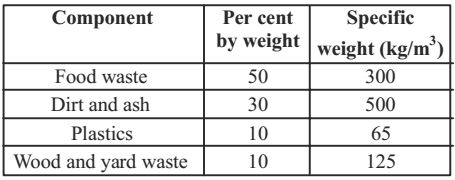## Municipal solid waste miscellaneous

#### Municipal Solid Waste

1. If the BOD3 of a waste water sample is 75 mg/l and reaction rate constant k (base e) is 0.345 per day, the amount of BOD remaining in the given sample after 10 days is

1. Lt = Lo e–kt
k = 0.345/d
BOD = Lo – Lt = Lo (1 – e–kt)
BOD3 = 75 = Lo(1 – e–0.345×3)
∴ Lo = 116.28 mg/l
∴ BOD10
Remaining BOD,
Lt = Lo e–kt
= 116.28 × e–10 × 0.345 = 3.69 mg/l.

##### Correct Option: C

Lt = Lo e–kt
k = 0.345/d
BOD = Lo – Lt = Lo (1 – e–kt)
BOD3 = 75 = Lo(1 – e–0.345×3)
∴ Lo = 116.28 mg/l
∴ BOD10
Remaining BOD,
Lt = Lo e–kt
= 116.28 × e–10 × 0.345 = 3.69 mg/l.

1. The composition of a certain MSW sample and specific weights of its various components are given below:Specific weight (kg/m³) of the MSW sample is

1. Specific weight of MSW = % of component × specific weight
= .5 × 300 + .3 × 500 + .1 × 65 + 0.1 × 125 = 319 kg/m³.

##### Correct Option: A

Specific weight of MSW = % of component × specific weight
= .5 × 300 + .3 × 500 + .1 × 65 + 0.1 × 125 = 319 kg/m³.

1. A wastewater sample has an initial BOD of 222 mg/L. The first order BOD decay coefficient is 0.4/day. The BOD consumed (in mg/L) in 5 days is

1. L5 = L0 [1 – e–k×t]
= 222 [1 – e–0.4×5] = 192 mg/L

##### Correct Option: B

L5 = L0 [1 – e–k×t]
= 222 [1 – e–0.4×5] = 192 mg/L

1. A coastal city produces municipal solid waste (MSW) with high moisture content, high organic materials, low calorific value and low inorganic materials. The most effective and sustainable option for MSW management in that city is

1. NA

##### Correct Option: A

NA

1. Determine the correctness or otherwise of the following Assertion (A) and the Reason (R).
(A): the crown of the outgoing larger diameter sewer is always matched with the crown of the incoming smaller diameter sewer.
Reason (R): It eliminates backing up of sewage in the incoming smaller diameter sewer.

1. NA

NA